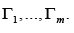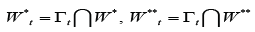# Sequential Decomposition Algorithm of Blocking the Selected Edges in the Digraph

Tsitsiashvili Gurami*

Institute for Applied Mathematics FEB RAS, Russia

Received Date: October 28, 2019;  Published Date: November 04, 2019

#### Abstract

In this paper, a protein network represented by a directed graph is considered. The problem of determining the minimum number of edges that break paths from the input proteins of the network to the output ones and passing through some subset of proteins in this network is analyzing. A sequential decomposition algorithm, basing on a selection of classes of cyclically equivalent nodes in the sub-graphs with dedicated subset of nodes and on a construction of some bipartite graph is suggesting. This algorithm increases significantly previous results obtained in [1,2].

Keywords: Cluster; Digraph; Sub-graph; Protein network; Connectivity component

#### Introduction

Our task is to determine the number of edges that allow blocking unwanted nodes in a directed graph (digraph). Consider a digraph G with a set of nodes U. Let a subset U/U of socalled undesirable nodes. Our task is to construct an algorithm for allocating a set of edges, removal of which from the digraph G leads to blocking (breaking) all paths entering the set U/ from the outside and leaving the set U/ outside.

This problem is NP- problem, but if you can somehow split the digraph G into sub graphs, it is possible to reduce the number of edges removed and to simplify the calculations by solving this problem for the selected sub-graphs. Indeed, denote p (denote q ) the total number of edges coming from the set U \U/ to the set U/ (coming from the set U/ to the set U \U/ . Then you can only delete edges whose number is less. Moreover, splitting the digraph into sub-graphs we reduce the total number of deleted edges.

#### Solution of the Problem

In , a digraph G/ is constructing of nodes from the set U/ and the edges between them.On the set of nodesU/in  the cyclic equivalence relationis introduced if in G/ there exists a cycle containing a,b (there exists a path from node a to node b ). Call the cyclic equivalence classes - clusters, extending the partial order relation ≥ to clusters, and draw between any clusters A, B an edge if such an edge exists in G/ .

Define the set of clusters W*(the set of clusters W** ) in which in the digraph G edges income from outside G/ (from which edges come outside G/ ). We draw generalized edges → from clusters A∈W* into clustersThe resulting bipartite digraph is denoting by Γ .

Eliminate the orientation of the edges → in the digraph Γ and select the components of connectivity in the resulting undirected graph, and then restore the orientation of the edges in them and get digraphsFor each digraph Γt defineand denote Pt,qtthe numbers of edges of the digraph G , entering from outside and leaving outside the digraph Γt respectively,t =1,...,m.

For each digraph, Γ twe remove those edges, which number is less,and we receive in equalityreinforcing the results of [1,2] significantly, because from the conditionit follows that the clustersare included in the same sub graph Γt of thedigraph Γ .

Supported by Russian Fund of Basic Research’s, project 17-07- 00177.

None.

#### Conflict of Interest

No conflict of interest.

Article Details
Citation
Keywords
Scroll to
Scroll to Top